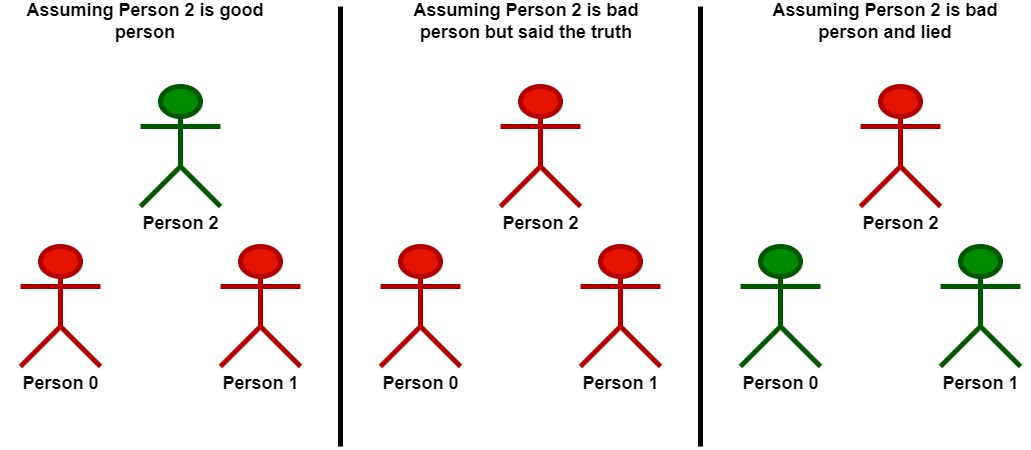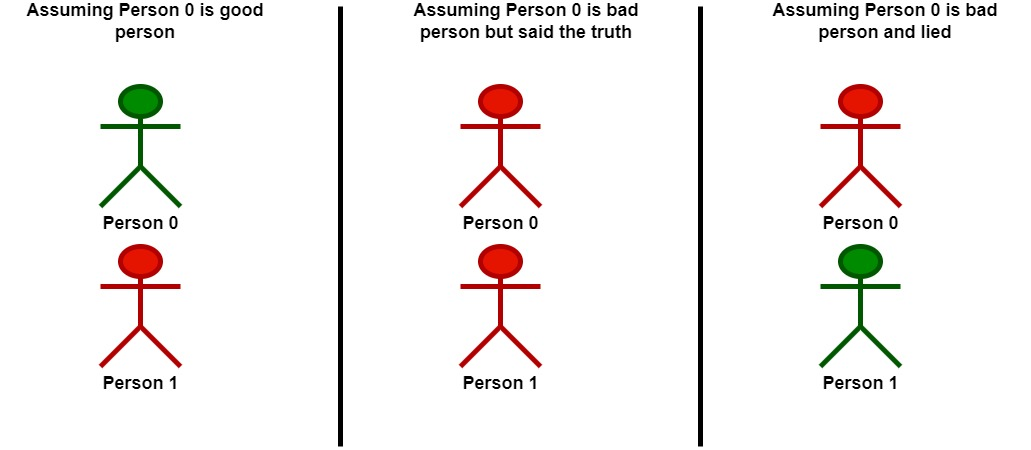##### Welcome to Subscribe On Youtube

Formatted question description: https://leetcode.ca/all/2151.html

# 2151. Maximum Good People Based on Statements (Hard)

There are two types of persons:

• The good person: The person who always tells the truth.
• The bad person: The person who might tell the truth and might lie.

You are given a 0-indexed 2D integer array statements of size n x n that represents the statements made by n people about each other. More specifically, statements[i][j] could be one of the following:

• 0 which represents a statement made by person i that person j is a bad person.
• 1 which represents a statement made by person i that person j is a good person.
• 2 represents that no statement is made by person i about person j.

Additionally, no person ever makes a statement about themselves. Formally, we have that statements[i][i] = 2 for all 0 <= i < n.

Return the maximum number of people who can be good based on the statements made by the n people.

Example 1:Input: statements = [[2,1,2],[1,2,2],[2,0,2]]
Output: 2
Explanation: Each person makes a single statement.
- Person 0 states that person 1 is good.
- Person 1 states that person 0 is good.
- Person 2 states that person 1 is bad.
Let's take person 2 as the key.
- Assuming that person 2 is a good person:
- Based on the statement made by person 2, person 1 is a bad person.
- Now we know for sure that person 1 is bad and person 2 is good.
- Based on the statement made by person 1, and since person 1 is bad, they could be:
- telling the truth. There will be a contradiction in this case and this assumption is invalid.
- lying. In this case, person 0 is also a bad person and lied in their statement.
- Following that person 2 is a good person, there will be only one good person in the group.
- Assuming that person 2 is a bad person:
- Based on the statement made by person 2, and since person 2 is bad, they could be:
- telling the truth. Following this scenario, person 0 and 1 are both bad as explained before.
- Following that person 2 is bad but told the truth, there will be no good persons in the group.
- lying. In this case person 1 is a good person.
- Since person 1 is a good person, person 0 is also a good person.
- Following that person 2 is bad and lied, there will be two good persons in the group.
We can see that at most 2 persons are good in the best case, so we return 2.
Note that there is more than one way to arrive at this conclusion.


Example 2:Input: statements = [[2,0],[0,2]]
Output: 1
Explanation: Each person makes a single statement.
- Person 0 states that person 1 is bad.
- Person 1 states that person 0 is bad.
Let's take person 0 as the key.
- Assuming that person 0 is a good person:
- Based on the statement made by person 0, person 1 is a bad person and was lying.
- Following that person 0 is a good person, there will be only one good person in the group.
- Assuming that person 0 is a bad person:
- Based on the statement made by person 0, and since person 0 is bad, they could be:
- telling the truth. Following this scenario, person 0 and 1 are both bad.
- Following that person 0 is bad but told the truth, there will be no good persons in the group.
- lying. In this case person 1 is a good person.
- Following that person 0 is bad and lied, there will be only one good person in the group.
We can see that at most, one person is good in the best case, so we return 1.
Note that there is more than one way to arrive at this conclusion.


Constraints:

• n == statements.length == statements[i].length
• 2 <= n <= 15
• statements[i][j] is either 0, 1, or 2.
• statements[i][i] == 2

Similar Questions:

## Solution 1. Bitmask Subset Traversal

Enumerate bitmask m from 1 to 2^N - 1. If the ith bit of m is 1/0, it means that person i is good/bad.

We can ignore bad persons’ statements because they can be either true or false. But good persons’ statements must be all correct.

To test if this bitmask m is valid, we go through all the good persons’ statements and see if there is any contradiction.

• // OJ: https://leetcode.com/problems/maximum-good-people-based-on-statements/
// Time: O(2^N * N^2)
// Space: O(1)
class Solution {
public:
int maximumGood(vector<vector<int>>& A) {
int N = A.size(), ans = 0;
auto valid = [&](int m) {
for (int i = 0; i < N; ++i) {
if (m >> i & 1) { // person i is good
for (int j = 0; j < N; ++j) { // test if there is any contradiction in the statements from person i
int good = m >> j & 1; // whether person j is good
if ((A[i][j] == 0 && good) || (A[i][j] == 1 && !good)) return false;
}
}
}
return true;
};
for (int m = 1; m < (1 << N); ++m) {
if (valid(m)) ans = max(ans, __builtin_popcount(m));
}
return ans;
}
};

• class Solution:
def maximumGood(self, statements: List[List[int]]) -> int:
cnt = 0
for i, s in enumerate(statements):
if (mask >> i) & 1:
for j, v in enumerate(s):
if v < 2 and ((mask >> j) & 1) != v:
return 0
cnt += 1
return cnt

############

# 2151. Maximum Good People Based on Statements
# https://leetcode.com/problems/maximum-good-people-based-on-statements/

class Solution:
def maximumGood(self, statements: List[List[int]]) -> int:
n = len(statements)

res = 0

for mask in range(1, 1 << n):
valid = True
good = 0

for j in range(n):
if mask & (1 << j) > 0:
good += 1
for k in range(n):
if statements[j][k] == 2: continue

if statements[j][k] == 0 and mask & (1 << k) > 0:
valid = False
break

elif statements[j][k] == 1 and mask & (1 << k) == 0:
valid = False
break

if valid:
res = max(res, good)

return res


• class Solution {
public int maximumGood(int[][] statements) {
int ans = 0;
}
return ans;
}

private int check(int mask, int[][] statements) {
int cnt = 0;
int n = statements.length;
for (int i = 0; i < n; ++i) {
if (((mask >> i) & 1) == 1) {
for (int j = 0; j < n; ++j) {
int v = statements[i][j];
if (v < 2 && ((mask >> j) & 1) != v) {
return 0;
}
}
++cnt;
}
}
return cnt;
}
}

• func maximumGood(statements [][]int) int {
n := len(statements)
check := func(mask int) int {
cnt := 0
for i, s := range statements {
if ((mask >> i) & 1) == 1 {
for j, v := range s {
if v < 2 && ((mask>>j)&1) != v {
return 0
}
}
cnt++
}
}
return cnt
}
ans := 0
}
return ans
}

func max(a, b int) int {
if a > b {
return a
}
return b
}

• function maximumGood(statements: number[][]): number {
const n = statements.length;
let cnt = 0;
for (let i = 0; i < n; ++i) {
if ((mask >> i) & 1) {
for (let j = 0; j < n; ++j) {
const v = statements[i][j];
if (v < 2 && ((mask >> j) & 1) != v) {
return 0;
}
}
++cnt;
}
}
return cnt;
}
let ans = 0;